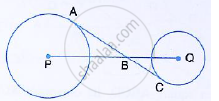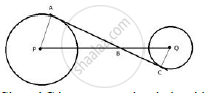Share

# In the Figure, Given Below, Ac is a Transverse Common Tangent to Two Circles with Centres P and Q and of Radii 6 Cm and 3 Cm Respectively. Given that Ab = 8 Cm, Calculate Pq. - Mathematics

Course

#### Question

In the figure, given below, AC is a transverse common tangent to two circles with centres P and Q and of radii 6 cm and 3 cm respectively.Given that AB = 8 cm, calculate PQ.

#### SolutionSince AC is tangent to the circle with center P at point A.
∴ ∠PAB =90°
Similarly, ∠QCB = 90°
In ΔPAB and ΔQCB
∠PAB = ∠OCB = 90°
∠PBA = ∠QBC (vertically opposite angles)
∴  Δ PAB  ˜  ΔQCB
⇒ "PA "/"QC"  = "PB"/"QB"         ……… (i)

Also in Rt. ΔPAB,
PB = sqrt(PA^2  + PB^2)
⇒ PB = sqrt(6^2 + 8^2)  = sqrt(36 + 64 ) = sqrt(100)  = 10 cm…….(ii)
From (i) and (ii)
6/3 =  (10 )/(QB)
⇒ QB = (3 × 10 )/6 = 5 cm
Now,
PQ = PB + QB = (10+5) cm = 15cm

Is there an error in this question or solution?